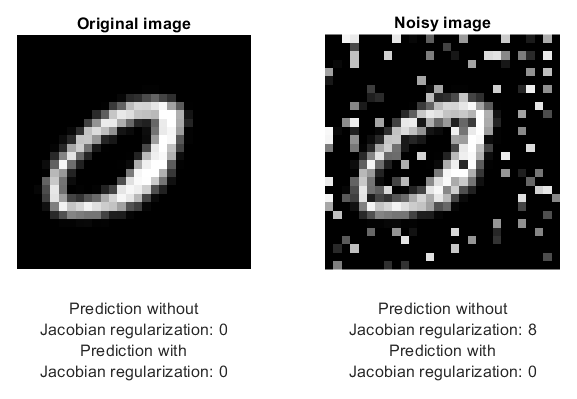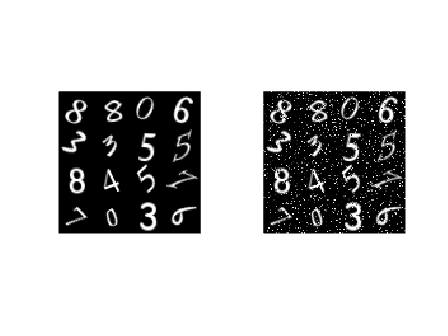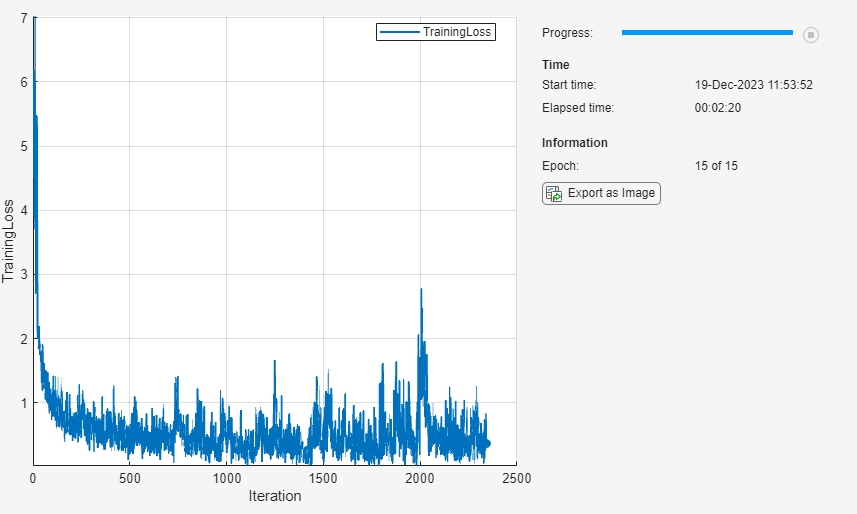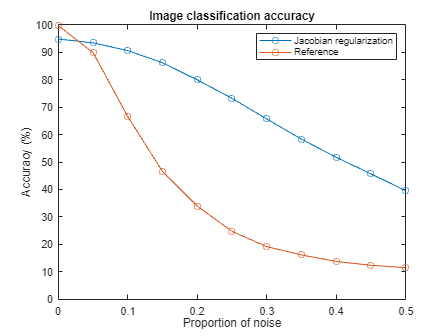# Train Robust Deep Learning Network with Jacobian Regularization

This example shows how to train a neural network that is robust to adversarial examples using a Jacobian regularization scheme .

Neural networks can be susceptible to a phenomenon known as adversarial examples , where very small changes to an input can cause it to be misclassified. These changes are often imperceptible to humans. Second-order methods such as Jacobian regularization have been shown to help increase the robustness of networks against adversaries .

For example, consider the figure below. On the left is an image of a zero, and on the right is the same image with white noise added to create an adversarial example. A network trained without Jacobian regularization classifies the original image of a zero correctly but misclassifies the adversarial example. In contrast, a network trained with Jacobian regularization classifies both the original and the noisy image correctly.This example shows how to:

1. Train a robust image classification network using a Jacobian regularization scheme.

2. Compare its predictions to a network trained without Jacobian regularization.

The `digitTrain4DArrayData` function loads the images and their digit labels. Create an `arrayDatastore` object for the images and the labels, and then use the `combine` function to make a single datastore that contains all of the training data.

```[XTrain,TTrain] = digitTrain4DArrayData; dsXTrain = arrayDatastore(XTrain,IterationDimension=4); dsTTrain = arrayDatastore(TTrain); dsTrain = combine(dsXTrain,dsTTrain);```

Determine the number of classes in the training data.

```classes = categories(TTrain); numClasses = numel(classes);```

Next, apply noise to the training images to create adversarial examples. Compare images from the training data with no noise and with noise affecting 10% of the pixels.

Select 16 images at random.

```numImages = size(XTrain,4); randompick = randperm(numImages,16); XOriginal = XTrain(:,:,:,randompick);```

Create the noisy images by setting a proportion of the pixels to a random grayscale value.

```noiseProp = 0.1; noise = rand(size(XOriginal)); idx = rand(size(XOriginal)) < noiseProp; XNoisy = XOriginal; XNoisy(idx) = noise(idx);```

Plot the original images next to the noisy images.

```I1 = imtile(XOriginal); I2 = imtile(XNoisy); figure; subplot(1,2,1) imshow(I1) subplot(1,2,2) imshow(I2)```### Define Network

Define the architecture of the network.

Specify an image input layer of the same size as the training images.

`imageInputSize = size(XTrain, 1:3)`
```imageInputSize = 1×3 28 28 1 ```
```layers = [ imageInputLayer(imageInputSize,Mean=mean(XTrain,4)) convolution2dLayer(5,20) batchNormalizationLayer reluLayer convolution2dLayer(3,20,Padding=1) batchNormalizationLayer reluLayer convolution2dLayer(3,20,Padding=1) batchNormalizationLayer reluLayer fullyConnectedLayer(10) softmaxLayer]; lgraph = layerGraph(layers);```

Create a `dlnetwork` object from the layer graph.

`dlnet = dlnetwork(lgraph);`

### Define Model Loss Function

Create the function `modelLoss`, listed at the end of the example, which takes a `dlnetwork` object, and a mini-batch of input data with corresponding labels, and returns the loss, the gradients of the loss with respect to the learnable parameters in the network, and the state of the network.

### Specify Training Options

Train for 15 epochs with a mini-batch size of 32.

```numEpochs = 15; miniBatchSize = 32;```

Specify the options for SGDM optimization. Specify a learning rate of 0.01 and a momentum of 0.9.

```learningRate = 0.01; momentum = 0.9;```

The Jacobian regularization ${\lambda }_{\mathrm{JR}}$is a hyperparameter that controls the effect of the Jacobian regularization term on the training of the network. If the coefficient is too large, then the cross-entropy term is not effectively minimized and the accuracy of the network classification is poor. If the coefficient is too small, the trained network does not have the expected robustness to white noise. For example, choose ${\lambda }_{\mathrm{JR}}=1$.

`jacobianRegularizationCoefficient = 1;`

### Train Model

Create a `minibatchqueue` object that processes and manages mini-batches of images during training. For each mini-batch:

• Use the custom mini-batch preprocessing function `preprocessMiniBatch` (defined at the end of this example) to convert the labels to one-hot encoded variables.

• Format the image data with the dimension labels `"SSCB"` (spatial, spatial, channel, batch). By default, the `minibatchqueue` object converts the data to `dlarray` objects with underlying type `single`. Do not add a format to the class labels.

• Train on a GPU if one is available. If a GPU is available, the `minibatchqueue` object converts each output to a `gpuArray` by default. Using a GPU requires Parallel Computing Toolbox™ and a supported GPU device. For information on supported devices, see GPU Support by Release (Parallel Computing Toolbox).

```mbq = minibatchqueue(dsTrain,... MiniBatchSize=miniBatchSize,... MiniBatchFcn=@preprocessMiniBatch,... PartialMiniBatch="discard",... MiniBatchFormat=["SSCB",""]);```

Initialize the training progress plot.

```figure; lineLossTrain = animatedline(Color=[0.85 0.325 0.098]); ylim([0 inf]) xlabel("Iteration") ylabel("Loss") grid on```

Initialize the velocity parameter for the SGDM solver.

`velocity = [];`

Train the network using a custom training loop.

For each epoch, shuffle the data and loop over mini-batches of data. For each mini-batch:

• Evaluate the model loss, gradients, and state using the `dlfeval` and `modelLoss` functions and update the network state.

• Update the network parameters using the `sgdmupdate` function.

• Display the training progress.

```iteration = 0; start = tic; % Loop over epochs. for epoch = 1:numEpochs % Reset and shuffle mini-batch queue. shuffle(mbq); while hasdata(mbq) iteration = iteration + 1; % Read mini-batch of data. [X, T] = next(mbq); % Evaluate the model loss, gradients and the network state using % dlfeval and the modelLoss function listed at the end of the example. [totalLoss, gradTotalLoss, state] = dlfeval(@modelLoss, dlnet, X, T, ... miniBatchSize, jacobianRegularizationCoefficient); dlnet.State = state; % Update the network parameters. [dlnet, velocity] = sgdmupdate(dlnet,gradTotalLoss,velocity,learningRate,momentum); % Plot the training progress. D = duration(0,0,toc(start),Format="hh:mm:ss"); addpoints(lineLossTrain,iteration,double(totalLoss)) title("Training with Jacobian regularization" + ", Epoch: " + epoch + ", Elapsed: " + string(D)) drawnow end end```Load a reference network, with the same layers, but trained without Jacobian regularization.

`dlnetReference = load("dlnetWithoutJacobian.mat").dlnetReference;`

### Test Model

Load the test data for a comparison test between the network trained with Jacobian regularization and the reference network.

```[XTest, TTest] = digitTest4DArrayData; classes = categories(TTest);```

Pass through test images that the networks have not seen before. With each pass, add noise affecting 0% to 50% of the pixels in increments of 5%.

```% Initialize test parameters and arrays. noiseProps = 0:0.05:0.5; % Prepare arguments for mini-batch queue. dsTTest = arrayDatastore(TTest); miniBatchSize = 5000; for i = 1:numel(noiseProps) % Load the noise proportion. noiseProp = noiseProps(i); fprintf("Testing robustness to noise proportion %1.2g\n", noiseProp) % Set a proportion of the pixels to a random grayscale value. noise = rand(size(XTest)); idx = rand(size(XTest)) < noiseProp; XNoisy = XTest; XNoisy(idx) = noise(idx); % Prepare mini-batch queue with noisy test data. dsXTest = arrayDatastore(XNoisy,IterationDimension=4); dsTest = combine(dsXTest,dsTTest); mbq = minibatchqueue(dsTest,... MiniBatchSize=miniBatchSize,... MiniBatchFcn=@preprocessMiniBatch,... MiniBatchFormat=["SSCB",""]); % Loop over batches to find predicted classifications. while hasdata(mbq) [XNoisy, T] = next(mbq); % Classify noisy data with the robust network. YPredNoisy = predict(dlnet, XNoisy); % Convert the predictions into labels. YPred = onehotdecode(YPredNoisy, classes, 1)'; TTestBatch = onehotdecode(T, classes, 1)'; % Evaluate accuracy of predictions. accuracyRobust(i) = mean(YPred == TTestBatch); % Classify noisy data with reference network. YPredNoisy = predict(dlnetReference, XNoisy); % Convert the predictions into labels. YPred = onehotdecode(YPredNoisy, classes, 1)'; % Evaluate accuracy of predictions. accuracyReference(i) = mean(YPred == TTestBatch); end end```
```Testing robustness to noise proportion 0 Testing robustness to noise proportion 0.05 Testing robustness to noise proportion 0.1 Testing robustness to noise proportion 0.15 Testing robustness to noise proportion 0.2 Testing robustness to noise proportion 0.25 Testing robustness to noise proportion 0.3 Testing robustness to noise proportion 0.35 Testing robustness to noise proportion 0.4 Testing robustness to noise proportion 0.45 Testing robustness to noise proportion 0.5 ```

Plot the results of the percentage accuracy of both networks against the proportion of the white noise.

Note that the network trained with Jacobian regularization has a slightly lower accuracy when the noise proportion is equal to 0 but achieves higher accuracy than the reference network when noise is added to the images.

```x = noiseProps'; figure; plot(x,accuracyRobust*100, "-o", x, accuracyReference*100, "-o") xlabel("Proportion of noise") ylabel("Accuracy (%)") xlim([0,0.5]); ylim([0,100]); title("Image classification accuracy") legend("Jacobian regularization", "Reference");```### Test Specific Example

Add noise affecting 15% of the pixels to the first test image, which contains the number 0. Plot both the original image and the image perturbed by the white noise. Use the network trained with Jacobian regularization and the reference network to classify the image.

```% Choose test image testchoice = 1; % Add noise, with a proportion of 0.15, to the first image. noise = rand(size(XTest(:,:,:,testchoice))); idx = rand(size(XTest(:,:,:,testchoice))) < 0.15; XNoisy = XTest(:,:,:,testchoice); XNoisy(idx) = noise(idx); % Convert to dlarray. XTest = dlarray(XTest(:,:,:,testchoice),"SSCB"); XNoisy = dlarray(XNoisy,"SSCB"); % Print true number classification disp("True digit label: " + char(TTest(testchoice)));```
```True digit label: 0 ```

Classify the original image by using the network trained with Jacobian regularization.

```YPredTestJR = predict(dlnet, XTest); YPredTestJR = onehotdecode(YPredTestJR, classes, 1)'; disp("Robust network classification of original image: " + char(YPredTestJR));```
```Robust network classification of original image: 0 ```

Classify the noisy image by using the network trained with Jacobian regularization.

```YPredNoisyJR = predict(dlnet, XNoisy); YPredNoisyJR = onehotdecode(YPredNoisyJR, classes, 1)'; disp("Robust network classification of noisy image: " + char(YPredNoisyJR));```
```Robust network classification of noisy image: 0 ```

Classify the original image by using the network trained without Jacobian regularization.

```YPredTest = predict(dlnetReference, XTest); YPredTestR = onehotdecode(YPredTest, classes, 1)'; disp("Reference network classification of original image: " + char(YPredTestR));```
```Reference network classification of original image: 0 ```

Classify the noisy image by using the network trained without Jacobian regularization.

```YPredNoisy = predict(dlnetReference, XNoisy); YPredNoisyR = onehotdecode(YPredNoisy, classes, 1)'; disp("Reference network classification of noisy image: " + char(YPredNoisyR));```
```Reference network classification of noisy image: 8 ```

Plot the original and noisy images and display the predictions given by each network.

```figure; I1 = extractdata(XTest(:,:,:,testchoice)); subplot(1,2,1) imshow(I1) title("Original image") xlabel({"Prediction without"; "Jacobian regularization: " + char(YPredTestR);... "Prediction with"; "Jacobian regularization: " + char(YPredTestJR)}) I2 = extractdata(XNoisy); subplot(1,2,2) imshow(I2) title("Noisy image") xlabel({"Prediction without"; "Jacobian regularization: " + char(YPredNoisyR);... "Prediction with"; "Jacobian regularization: " + char(YPredNoisyJR)})```### Model Loss Function

The goal of Jacobian regularization is to penalize large changes of the prediction $\mathit{y}$ with respect to small changes in the input $\mathit{x}$. Doing so makes the network more robust to input data polluted by noise. The Jacobian $\mathit{J}$ encodes the change of prediction with respect to the input by containing the partial derivatives of $\mathit{y}$ with respect to $\mathit{x}$.

`$\mathit{J}=\left[\begin{array}{ccc}\frac{\partial \text{\hspace{0.17em}}{\mathit{y}}_{1}}{\partial \text{\hspace{0.17em}}{\mathit{x}}_{1}}& \cdots & \frac{\text{\hspace{0.17em}}\partial \text{\hspace{0.17em}}{\mathit{y}}_{1}}{\partial \text{\hspace{0.17em}}{\mathit{x}}_{\mathit{n}}}\\ ⋮& \ddots & ⋮\\ \frac{\text{\hspace{0.17em}}\partial \text{\hspace{0.17em}}{\mathit{y}}_{\mathit{m}}}{\partial \text{\hspace{0.17em}}{\mathit{x}}_{1}}& \cdots & \frac{\text{\hspace{0.17em}}\partial \text{\hspace{0.17em}}{\mathit{y}}_{\mathit{m}}}{\partial \text{\hspace{0.17em}}{\mathit{x}}_{\mathit{n}}}\end{array}\right]$`

Jacobian regularization is achieved by adding the Frobenius norm of the Jacobian to the loss function that is subsequently minimized when you train the network. However, the Jacobian can be expensive to compute, requiring $\mathit{m}$ backward passes through the network, where $\mathit{m}$ is the size of the output $\mathit{y}$. Therefore, instead of computing the full Jacobian, an approximation to the Frobenius norm of the Jacobian $\left(\mathrm{JR}\right)$ is computed as follows :

$\text{\hspace{0.17em}}{‖\text{\hspace{0.17em}}\mathit{J}\text{\hspace{0.17em}}‖}_{\mathit{F}}^{2}=\mathrm{tr}\left(\mathit{J}\text{\hspace{0.17em}}{\mathit{J}}^{\top \text{\hspace{0.17em}}}\right)={\mathit{E}}_{\mathit{v}\sim {\mathit{N}\left(0,{\mathit{I}}_{\mathit{m}}\right)}^{}\text{\hspace{0.17em}}}{\mathit{v}}^{\top \text{\hspace{0.17em}}}{\mathrm{JJ}}^{\top \text{\hspace{0.17em}}}\mathit{v}\approx \frac{1}{{\mathit{m}}_{\mathrm{proj}}\text{\hspace{0.17em}}}{\sum }_{\mathit{k}=1}^{{\mathit{m}}_{\mathrm{proj}}}$$‖\text{\hspace{0.17em}}{\mathit{v}}_{\mathit{k}}^{\top \text{\hspace{0.17em}}}\mathit{J}\text{\hspace{0.17em}}‖{\text{\hspace{0.17em}}}_{2}^{2}\text{\hspace{0.17em}}=\frac{1}{{\mathit{m}}_{\mathrm{proj}}}$$\sum _{\mathit{k}=1}^{{\mathit{m}}_{\mathrm{proj}}}$$‖\text{\hspace{0.17em}}{\nabla }_{\mathit{x}\text{\hspace{0.17em}}}\left[{\mathit{v}}_{\mathit{k}\text{\hspace{0.17em}}}^{\top \text{\hspace{0.17em}}}\mathit{y}\right]{‖}_{2}^{2}$.

where ${\mathit{v}}_{\mathit{k}\text{\hspace{0.17em}}}\sim \mathit{N}\left(0,{\mathit{I}}_{\mathit{m}}\right)$ is a draw from the standard Normal distribution and ${I}_{m}$ is is the m-by-m identity matrix. This can be implemented as follows:

`$\mathrm{JR}=0$`

Choose a mini-batch size $\mathit{B}$

For $\mathit{i}=1,\dots ,{\mathit{m}}_{\mathrm{proj}}$

1. Sample a random vector $\mathit{v}\sim \mathit{N}\left(0,{\mathit{I}}_{\mathit{m}}\right)$

2. Normalize the random vector $\mathit{v}=\frac{\mathit{v}}{‖\text{\hspace{0.17em}}\mathit{v}\text{\hspace{0.17em}}‖{\text{\hspace{0.17em}}}_{2}}$

3. Compute the derivative ${\nabla }_{\mathit{x}}\left({\mathit{v}}^{\top \text{\hspace{0.17em}}}\mathit{y}\right)$

4. $\mathrm{JR}=\mathrm{JR}+\frac{\mathit{m}{‖\text{\hspace{0.17em}}\nabla }_{\mathit{x}}\left({\mathit{v}}^{\top \text{\hspace{0.17em}}}\mathit{y}\right)‖{\text{\hspace{0.17em}}}_{2}^{2}\text{\hspace{0.17em}}}{{\mathit{B}\text{\hspace{0.17em}}\mathit{m}}_{\mathrm{proj}\text{\hspace{0.17em}}}}$

The gradient of the vector inner product requires one backward pass to compute. So, this approximation requires only ${\mathit{m}}_{\mathrm{proj}}$ backward passes to compute, and in practice, ${\mathit{m}}_{\mathrm{proj}}=1$.

In this example, the cross-entropy between the predicted classification $\mathit{y}$ and the true classification $\mathit{z}$ is used, resulting in the loss function

`$\mathrm{loss}=\mathrm{crossentropy}+\frac{{\lambda }_{\mathrm{JR}}}{2}\mathrm{JR}$`

where ${\lambda }_{\mathrm{JR}}$ is the Jacobian regularization coefficient. The approximation of the Frobenius norm of the Jacobian requires taking gradients with respect to $\mathit{x}$, and the training phase requires taking gradients of the loss with respect to the parameters. These calculations require support for second-order automatic differentiation.

The `modelLoss` function is used during the training of the network. It takes as input the network, the input data `X`, their respective classifications `T`, the mini-batch size `miniBatchSize`, and the Jacobian regularization coefficient `jacobianRegularizationCoefficient`. The function returns the total loss `totalLoss`, the gradient of the loss with respect to the network parameters `gradTotalLoss`, and the state of the network `state`. To compute the approximation to the norm of the Jacobian, a derivative of a vector-vector dot product is taken. Since a second order derivative is necessary to compute the gradient of the loss function with respect to the network parameters, you must set the option `EnableHigherDerivatives` to `true` when calling the function `dlgradient`.

```function [totalLoss, gradTotalLoss, state] = modelLoss(net, X, T, miniBatchSize, jacobianRegularizationCoefficient) % Find prediction and loss. [Z,state] = forward(net, X); loss = crossentropy(Z, T); numClasses = size(Z,1); numProjections = 1; regularizationTerm = 0; % Compute Jacobian term and its gradient. for i = 1:numProjections % Sample a matrix whose elements are drawn from the standard Normal distribution. rndarray = randn(numClasses, miniBatchSize); % Normalize the columns of the random matrix. rndarray = normc(rndarray); % Compute the vector-vector product. vectorproduct = rndarray(:)' * Z(:); % Compute the gradient of the vector-vector product. Since another % derivative will be taken, set EnableHigherDerivatives to true. vectorJacobianTerm = dlgradient(vectorproduct, X, EnableHigherDerivatives=true); % Multiply by necessary constants to obtain approximation of the % Frobenius norm of the Jacobian. regularizationTerm = regularizationTerm + numClasses*sum(vectorJacobianTerm.^2,"all") /(numProjections*miniBatchSize); end totalLoss = loss + jacobianRegularizationCoefficient/2 * regularizationTerm; % Calculate the gradient of the loss. gradTotalLoss = dlgradient(totalLoss, net.Learnables); end ```

### Mini-Batch Preprocessing Function

The `preprocessMiniBatch` function preprocesses the data using the following steps:

1. Extract the image data from the incoming cell array and concatenate into a numeric array. Concatenating over the fourth dimension adds a third dimension to each image, to be used as a singleton channel dimension.

2. Extract the label data from the incoming cell array and concatenate the data into a categorical array along the second dimension.

3. One-hot encode the categorical labels into numeric arrays. Encoding the labels into the first dimension produces an encoded array that matches the shape of the network output.

```function [X, T] = preprocessMiniBatch(XCell,TCell) % Extract image data from cell and concatenate X = cat(4,XCell{1:end}); % Extract label data from cell and concatenate T = cat(2,TCell{1:end}); % One-hot encode labels T = onehotencode(T,1); end```

### References

1. Hoffman, Judy, Daniel A. Roberts, and Sho Yaida. “Robust Learning with Jacobian Regularization.” Preprint, submitted August 7, 2019. https://arxiv.org/abs/1908.02729.

2. Szegedy, Christian, Wojciech Zaremba, Ilya Sutskever, Joan Bruna, Dumitru Erhan, Ian Goodfellow, and Rob Fergus. “Intriguing Properties of Neural Networks.” Preprint, submitted February 19, 2014. http://arxiv.org/abs/1312.6199.

3. Ma, Avery, Fartash Faghri, and Amir-Massoud Farahmand. “Adversarial Robustness through Regularization: A Second-Order Approach.” Preprint, submitted, April 3, 2020. http://arxiv.org/abs/2004.01832.

4. Goodfellow, Ian J., Jonathon Shlens, and Christian Szegedy. “Explaining and Harnessing Adversarial Examples.” Preprint, submitted, March 20, 2015. http://arxiv.org/abs/1412.6572.